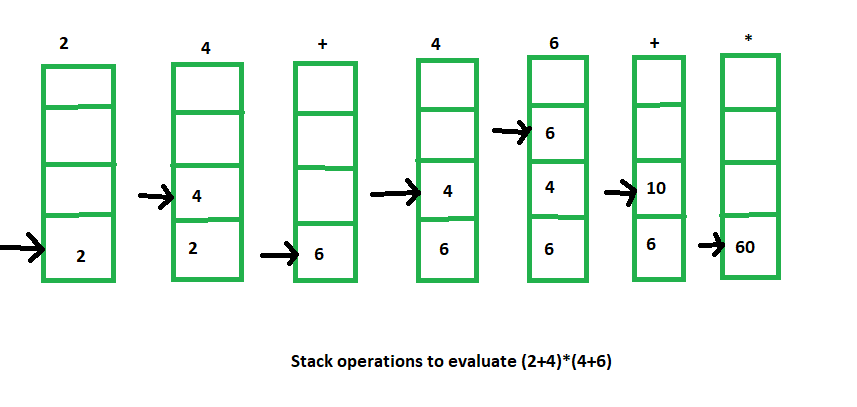# Arithmetic Expression Evalution

The stack organization is very effective in evaluating arithmetic expressions. Expressions are usually represented in what is known as Infix notation, in which each operator is written between two operands (i.e., A + B). With this notation, we must distinguish between ( A + B )*C and A + ( B * C ) by using either parentheses or some operator-precedence convention. Thus, the order of operators and operands in an arithmetic expression does not uniquely determine the order in which the operations are to be performed.

1. Polish notation (prefix notation) –
It refers to the notation in which the operator is placed before its two operands . Here no parentheses are required, i.e.,

`+AB `
2. Reverse Polish notation(postfix notation) –
It refers to the analogous notation in which the operator is placed after its two operands. Again, no parentheses is required in Reverse Polish notation, i.e.,

`AB+ `

Stack organized computers are better suited for post-fix notation then the traditional infix ntation. Thus the infix notation must be converted to the post-fix notation. The conversion from infix notation to post-fix notation must take into consideration the operational hierarchy.

There are 3 levels of precedence for 5 binary operators as given below:

```Highest: Exponentiation (^)
Next highest: Multiplication (*) and division (/)
Lowest: Addition (+) and Subtraction (-) ```

For example –

```Infix notation: (A-B)*[C/(D+E)+F]
Post-fix notation: AB- CDE +/F +* ```

Here, we first perform the arithmetic inside the parentheses (A-B) and (D+E). The division of C/(D+E) must done prior to the addition with F. After that multiply the two terms inside the parentheses and bracket.

Now we need to calculate the value of these arithmetic operations by using stack.

The procedure for getting the result is:

1. Convert the expression in Reverse Polish notation( post-fix notation).
2. Push the operands into the stack in the order they are appear.
3. When any operator encounter then pop two topmost operands for executing the operation.
4. After execution push the result obtained into the stack.
5. After the complete execution of expression the final result remains on the top of the stack.

For example –

```Infix notation: (2+4) * (4+6)
Post-fix notation: 2 4 + 4 6 + *
Result: 60 ```

The stack operations for this expression evaluation is shown below:Attention reader! Don’t stop learning now. Get hold of all the important DSA concepts with the DSA Self Paced Course at a student-friendly price and become industry ready.

My Personal Notes arrow_drop_upCheck out this Author's contributed articles.

If you like GeeksforGeeks and would like to contribute, you can also write an article using contribute.geeksforgeeks.org or mail your article to contribute@geeksforgeeks.org. See your article appearing on the GeeksforGeeks main page and help other Geeks.

Please Improve this article if you find anything incorrect by clicking on the "Improve Article" button below.

Article Tags :
Practice Tags :

8

Please write to us at contribute@geeksforgeeks.org to report any issue with the above content.